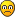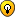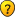## A BIT OF MATHS

Anything goes, but keep it seemly...
& my 1st guess is 3 volumes each 3 inches thick

to me to get to this conclusion quickly means that it is completely WRONGFor a perfect result 4+100 = 104 which makes it 2 volumes for the 1st worm & 10 volumes for the total number of volumes each being 1 inch thick (however perfect this may be, the covers are 0 inches thick)

tarektarek

Posts: 3351
Joined: 05 January 2006

tarek wrote:& my 1st guess is 3 volumes each 3 inches thick

to me to get to this conclusion quickly means that it is completely WRONGFor a perfect result 4+100 = 104 which makes it 2 volumes for the 1st worm & 10 volumes for the total number of volumes each being 1 inch thick (however perfect this may be, the covers are 0 inches thick)

tarek

I'm going to toss my 2 cents in here also... I've been struggling with the cover thickness too. I figure if a book's thickness is P+2C, where P is the page thickness and C is 1 cover thickness, then worm 1 travels 2P+2C - inside first book's first cover, through b1 pages, through b1 end cover, through b2 start cover, through b2 pages. Now how to make that fit into any other equation, I don't know.

So no solution to that yet, but here's another tricky bit I spotted:

the first worm eats from top left of page 1 to bottom right of page 100. but there's 50 leaves, so page 100 is reversed from page 1, and so bottom right of page 100 is really the same edge as top left of page 1, so instead of 2x^2 + 2<thickness>^2 == 11^2 it's really only x^2 +<thickness>^2 == 121.

Fortunately this doesn't change the path lengths for the other two... the longer is still 4 inches greater than the shorter no matter which way around.
EnderGT

Posts: 69
Joined: 19 February 2008

EnderGT wrote:but here's another tricky bit I spotted:

the first worm eats from top left of page 1 to bottom right of page 100. but there's 50 leaves, so page 100 is reversed from page 1, and so bottom right of page 100 is really the same edge as top left of page 1
I didn't spot that... That probably changes things a bittarektarek

Posts: 3351
Joined: 05 January 2006

This is similar to a problem I was presented in the stone age while in high school.

The first worm eats diagonally through the front cover of the first book and the back cover of the last book.

The other two worms eat through the same covers ... plus the covers and pages of the remaining volumes ... using different routes to get to the same destination.

Requiring integral inches in the solution seems to force right triangles with integer dimensions. Thus, only integer dimensions to (a**2 + b**2 = c**2) will work.
daj95376
2014 Supporter

Posts: 2624
Joined: 15 May 2006

daj95376 wrote:This is similar to a problem I was presented in the stone age while in high school.

The first worm eats diagonally through the front cover of the first book and the back cover of the last book.

The other two worms eat through the same covers ... plus the covers and pages of the remaining volumes ... using different routes to get to the same destination.

Requiring integral inches in the solution seems to force right triangles with integer dimensions. Thus, only integer dimensions to (a**2 + b**2 = c**2) will work.

the problem here is that there is no integer value combination that solves X^2 + Y^2 == 121.

So let's assume that I'm wrong about that bit of the puzzle, and work on the rest.

2*X^2 + Y^2 = 121 can be solved with 7*6*6. Since height and width must be the same, that makes the books 6*6. Since there are 2 books, the worm traveled through 3.5 inches of each book. Since the book's thickness must be odd, this leaves us with 1.5 inches of cover that the worm didn't travel through, for a total book thickness of 5 inches. Edit: This could also be 3.5 inches of cover for a book thickness of 7 inches if you allow 0 page thickness. Since book thickness must be odd, these are the only solutions. However, this solution doesn't change the situation below.

Now B (distance of worm 3) - A (distance of worm 2) == 4, but B^2 - A^2 == 36. This has no integer solution.

Edit: my reasoning for A and B:
A^2 = X^2 + (N * M - 3)^2
B^2 = X^2 + X^2 + (N * M - 3)^2
where M is the thickness of the books. Since the difference between these equations is the second X^2 term, and we know X to be 6 (from the above step), then B^2 - A^2 == 36.

So the solution, at least for the height and width of the books, must not be an integer.
EnderGT

Posts: 69
Joined: 19 February 2008

Ok, attacking this from the other direction.

Let A be the length of the path of worm 2 and B be the length of the path of worm 3 such that B - A == 4.

A can be no shorter than 11 inches, because that is the length of worm 1's travels in 2 books and so we know that is the lower bound.

B^2 - A^2 will give us the width/height of the books (squared). We know this must be less than 121, else the path length for worm 1 would exceed 11 inches.

That gives us a maximum of A=13 and B=17 (Delta = 120).

Since we know the travel lengths are whole numbers, our choices are thus

A == 11, B == 15, X == 10.19, W = 4.123105
A == 12, B == 16, X == 10.58, W = 3
A == 13, B == 17, X == 10.95, W = 1

Where W is the distance traveled by worm 1 along the spines of the books.

So worm 1 traveled either 2.0615525 in each book, giving us 3 inch books with 0.9384475 inch covers and 1.123105 inches of pages, or worm 1 traveled 1.5 inches per book, giving us 3 inch books with 1.5 inch covers and 0 inch pages, or worm 1 traveled 0.5 inches in each book, giving us 1 inch books with 0.5 inch covers and 0 inch pages.

I'm going to assume that 0 inch pages are not allowed, and so I'm going to say that there are 2 books that are 3 inches wide.

Edit: The problem with this answer is that the original question stated that the worms traveled in different directions, and so the path of worm 2 cannot be the same as the path of worm 1. Back to the drawing board...
Last edited by EnderGT on Mon Mar 31, 2008 7:04 pm, edited 1 time in total.
EnderGT

Posts: 69
Joined: 19 February 2008

This problem is really bugging me. Given all of the restrictions, there doesn't seem to be a solution.

1. The books are square, and so their height and width are equal. The height and width of the books must be less than 11, otherwise the length of worm 1's path would be greater than 11.
2. If A is the distance traveled by worm 2, B is the distance traveled by worm 3, and X is the width/height of the books, then A^2 + X^2 == B^2. Since X must be less than 11, B^2 - A^2 < 121. With B - A == 4, this gives us a limited set of choices for A and B.
4. Since all three path lengths must be different, we know there must be more than 2 books, else the path of worm 1 would be the same as one of the other worms.
5. Since Worms 2 and 3 travel to the last book, we know that the longer path length must be longer than 11. Whether or not the shorter is longer than 11 depends on which route worm 1 takes - whether it's route is calculated by 2*X^2 + W^2 == 121 or X^2 + W^2 == 121 (where W is the distance traveled parallel to the spine of the books).
6. An examination of the possible values for A and B, and, more importantly, the resulting values for X and W, eliminates 2*X^2 + W^2 = 121 from possibility. This forces the shorter path to also be longer than 11.
7. The remaining values for A and B result in books that have 0.5 or 1.5 inch thick covers but no page thickness.

Have I missed something? Have I applied a restriction that isn't really there?
EnderGT

Posts: 69
Joined: 19 February 2008

Hate to see you guys struggling.My suggestion: go to your local library, check out how they arrange the multi-volume books on the shelfs.Triple click below to see the answer I wrote:Total number of volumes = 4
Thickness of each volume = 3 inches
Thickness of each cover = 1/2 inch
Thickness of 50 leaves = 2 inches
Height/width of each leaf = sqrt(120) inches
Distances of the paths of the 3 worms = 11, 13, 17 inches
udosuk

Posts: 2698
Joined: 17 July 2005

I had a look at the question again (I missed that it says bottom corners) rather than corner.

I will recheck how I answer it.

udosuk, long time no see .....your journey lengths are not 4 inches different

tarektarek

Posts: 3351
Joined: 05 January 2006

tarek wrote:your journey lengths are not 4 inches different.

Yes they are. Use a calculator to verify this:

Triple click below to see what I wrote:17-13=4

11 is the journey length of the first worm (as given in the question).

Triple click below to see the extra hint I wrote:Which cover of volume 1 is next to which cover of volume 2?udosuk

Posts: 2698
Joined: 17 July 2005

udosuk wrote:Yes they are. Use a calculator to verify this:
17-13=4You are right, the question was referring to other 2 worms journey lengths. I've already said that I missed that prtion altogether & therrfore the questioning that followed.

It sounded strange at first (why 3 if worm 2 & 3 are doing the same thing)... it turned out that each went to a different bottom corner.

So we have 3 straight lines now ....

that should make it easier

tarektarek

Posts: 3351
Joined: 05 January 2006

tarek wrote:that should make it easier

Blah... So once again it's poor English understanding of the answerer (or poor English writing of the questioner) gets the honour to be blamed...Advice: next time make sure you carefully read through the whole question 3 times (more if you still don't get the clear picture) before you attempt to answer.

Advice 2: go to this thread and attempt to answer those 3 mini puzzles as an exercise!udosuk

Posts: 2698
Joined: 17 July 2005

udosuk wrote:Advice: next time make sure you carefully read through the whole question 3 times (more if you still don't get the clear picture) before you attempt to answer.
A mug of strong coffee should also be included. it would also help if you were a bookworm yourself.

Was there a hint of you doubting me getting to the correct solution udosuktarektarek

Posts: 3351
Joined: 05 January 2006

udosuk wrote:Advice: next time make sure you carefully read through the whole question 3 times (more if you still don't get the clear picture) before you attempt to answer.

Wow, no need to be such a jerk about it...udosuk wrote:My suggestion: go to your local library, check out how they arrange the multi-volume books on the shelfs.

This does seem to be my error, though. Once I switch the order of the books around, the math above works to give me a choice of 0.5 inch covers or 1.5 inch covers. Using A=13 gives me 7 inches of travel along the spines of the books. Take away the last and first covers gives me 6 inches. Since book sizes must be odd, the books must be 3 inches thick. So the final answer works out to 4 3-inch books.

So I had the math right but the order of books wrong. Blah.
EnderGT

Posts: 69
Joined: 19 February 2008

& udosuk,
WE ARE not HATING YOU because you got the answer right.tarek

Posts: 3351
Joined: 05 January 2006

PreviousNext